## How to use SOH CAH TOA: Geometry

Welcome back to Mathsux! This week, we’re going to go over how to find missing angles and side lengths of right triangles by using trigonometric ratios (sine, cosine, and tangent) (aka how to use SOH CAH TOA).  Woo hoo! These are the basics of right triangle trigonometry, and provides the base for mastering so many more interesting things in trigonometry! So, let’s get to it!

Also, if you have any questions about anything here, don’t hesitate to comment below or watch the video below. Also, don;r forget to subscribe to Math Sux for FREE math videos, lessons, and practice questions every week. Happy calculating! 🙂

## How to use SOH CAH TOA?

Trigonometric Ratios (more commonly known as Sine, Cosine, and Tangent) are ratios that naturally exist within a right triangle.  This means that the sides and angles of a right triangle are in proportion within itself.  It also means that if we are missing a side or an angle, based on what we’re given, we can probably find it!

Let’s take a look at what Sine, Cosine, and Tangent are all about!

Now let’s see how we can apply trig ratios when there is a missing side or angle in a right triangle!

Now for another type of question; using trig functions to find missing angles, let’s take a look:

Try the following Practice Questions on your own!

Solutions:

Still got questions?  No problem! Check out the video the same examples outlined above and happy calculating! 🙂

Need to brush up on special right triangles? Check out this posts on the 45 45 90 special triangles here!

## Median of a Trapezoid Theorem: Geometry

Hi everyone and welcome to Math Sux! In this post, we are going to look at how to use and applythe median of a trapezoid theorem. Thankfully, it is not a scary formula, and one we can easily master with a dose of algebra. The only hard part remaining, is remembering this thing! Take a look below to see a step by step tutorial on how to use the median of a trapezoid theorem and check out the practice questions at the end of this post to truly master the topic. Happy calculating! 🙂

*If you haven’t done so, check out the video that goes over this exact problem, also please don’t forget to subscribe!

Step 1:  Let’s apply the Median of a Trapezoid Theorem to this question!  A little rusty?  No problem, check out the Theorem below.

Median of a Trapezoid Theorem: The median of a trapezoid is equal to the sum of both bases.Step 2: Now that we found the value of x , we can plug it back into the equation for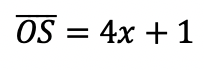median,  to find the value of median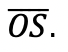Want more practice?  Your wish is my command! Check out the practice problems below:

Practice Questions:

1.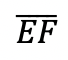is the median of trapezoid ABCDEF, find the value of the median, given the following:2.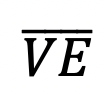is the median of trapezoid ACTIVE, find the value of the median, given the following:3.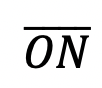is the median of  trapezoid DRAGON, find the value of the median, given the following:

4.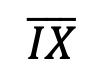is the median of trapezoid MATRIX, find the value of the median, given the following:

Solutions:

Need more of an explanation?  Check out the detailedand practice problems. Happy calculating! 🙂

## Completing the Square: Algebra

Want to learn the ins and out of completing the square?  Then you’ve come to the right place! Learn how to Complete the Square step by step in the video and article below, then try the practice problems at the end of this post to truly master the topic! If you’re looking for more on completing the square, check out this post here. Happy Calculating! 🙂 Check out the video below for an in-depth look at completing the square: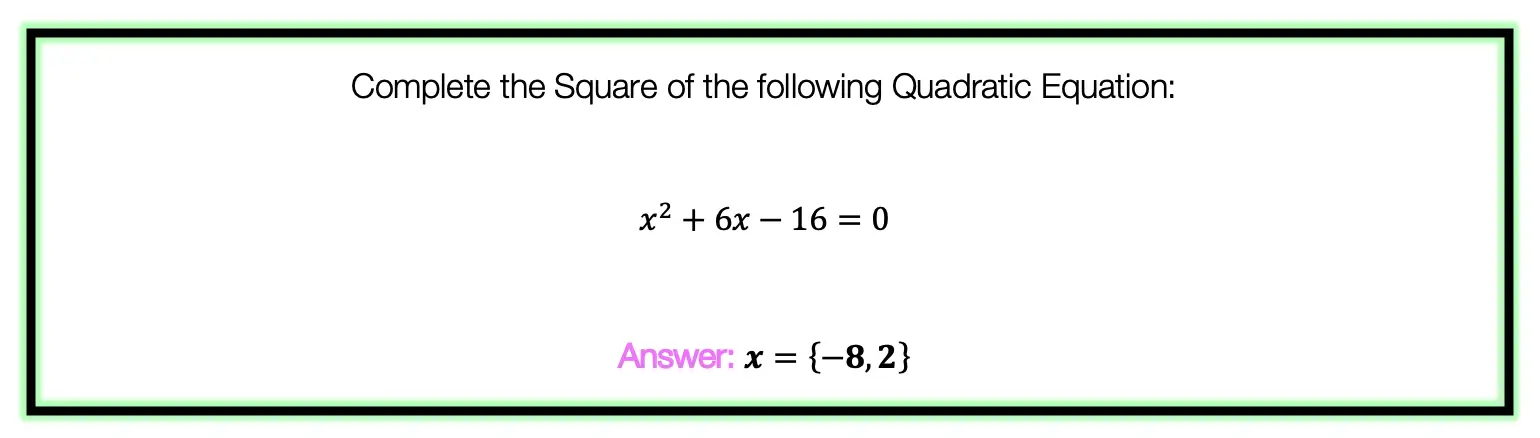To answer this question, there are several steps we must follow including: Step 1: Move the whole number, which in this case is 16, to the other side of the equation.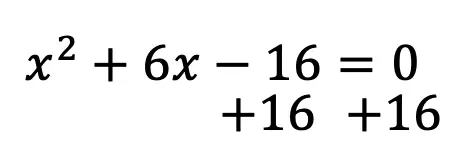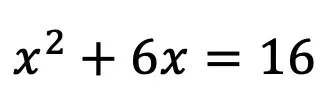Step 2: Make space for our new number on both sides of the equation.  This number is going to be found by using a particular formula shown below: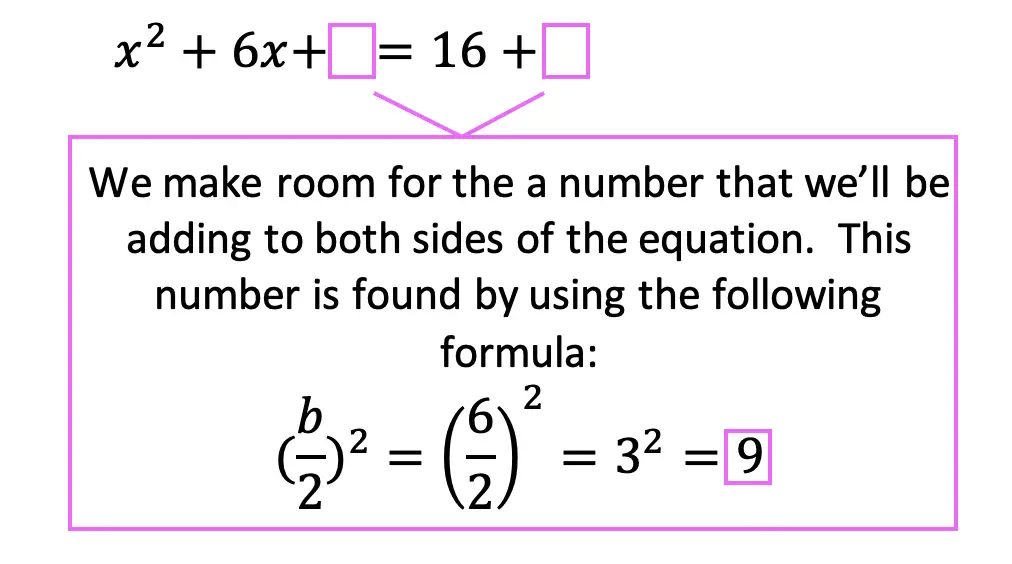Step 3: Add the number 9 to both sides of the equation, which we found using our formula.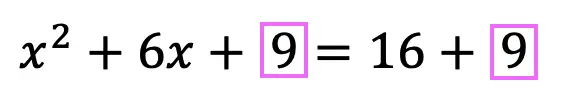Step 4: Combine like terms on the right side of the equation, adding 16+9 to get 25.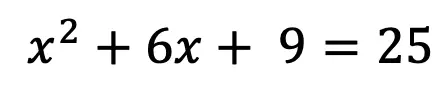Step 5: Now, we need to re-write the left side of the equation using the following formula.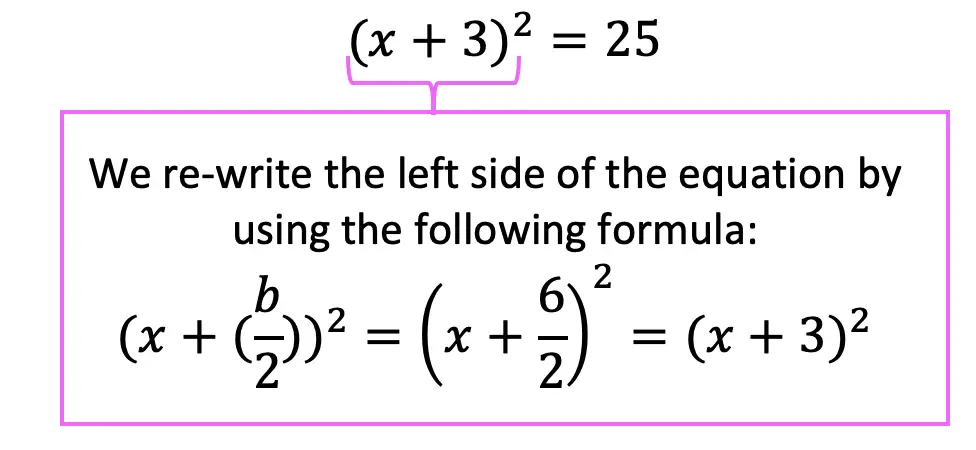Step 6: Finally, we solve for x by taking the positive and negative square root to get the following answer and solve for two different equations: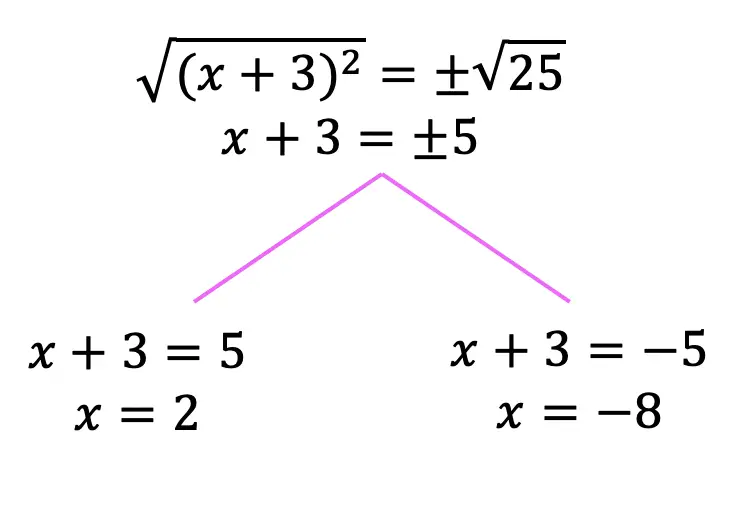Practice Questions: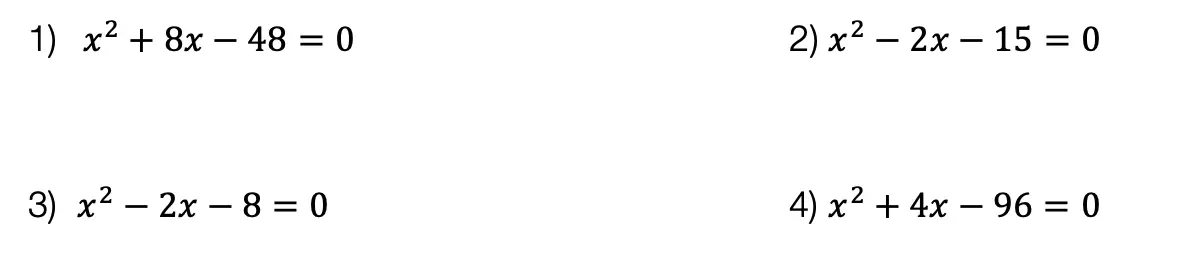Solutions: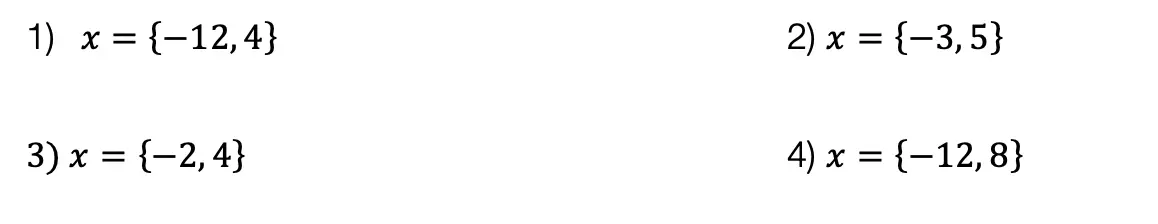Want more Mathsux?  Don’t forget to check out our Youtube channel and more below! And if you have any questions, please don’t hesitate to comment below. Happy Calculating! 🙂

Need more of an explanation?  Check out why we complete the square in the first place here ! 🙂

## Area of a Sector: Geometry

Hi math friends, has anyone been cooking more during quarantine?  We all know there is more time for cookin’ and eatin’ cakes but have you ever been curious about the exact amount of cake you are actually eating?! Well, you’re in luck because today we are going to go over how to find the area of a piece of cake, otherwise known as the Area of a Sector!

Now, we’ll all be able to calculate just how much we are overdoing it on that pie! Hopefully, everyone is eating better than I am (I should really calm down on the cupcakes).  Ok, now to our question:

*Also, If you haven’t done so, check out the video that goes over this exact problem, and don’t forget to subscribe!

## Explanation:

How do I answer this question?

We must apply/adjust the formula for the area of a circle to find the area of the blue shaded region otherwise known as the sector of this circle.

How do we do this?

Before we begin let’s review the formula for the area of a circle. Just a quick reminder of what each piece of the formula represents:

Step 1: Now let’s fill in our formula, we know the radius is 5, so let’s fill that in below:

Step 2: Ok, great! But wait, this is for a sector; We need only a piece of the circle, not the whole thing.  In other words, we need a fraction of the circle. How can we represent the area of the shaded region as a fraction?

Well, we can use the given central angle value,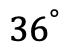, and place it over the whole value of the circle,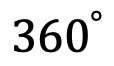. Then multiply that by the area of the entire circle. This will give us the value we are looking for!

Step 3: Multiply and solve!

Ready for more? Try solving these next few examples on your own to truly master area of a sector!

## Practice Questions:

Find the area of each shaded region given the central angle and radius for each circle:

## Solutions:

What do you think of finding the area of sector? Are you going to measure the area of your next slice of pizza?  Do you have any recipes to recommend?  Let me know in the comments and happy calculating! 🙂

Looking for more about circles? Check out this post on the circle formula here!